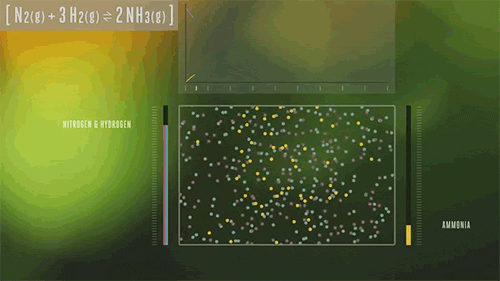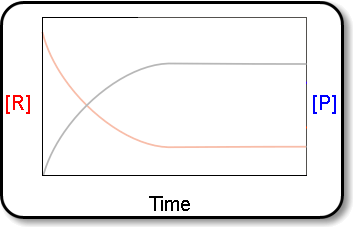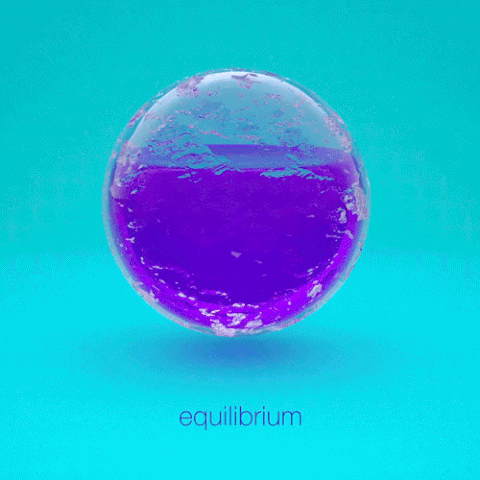Search

# Equilibrium

Updated: Oct 23, 2019

The characteristics of equilibrium are:It is dynamic, e.g can go in both directions.

It is only achieved in closed systems.

The concentration is constant.

There is no macroscopic directions.

At equilibrium the rate of the forward and the backward reaction is equal to the rate of the backward reaction. Reactants ⇆ Products.

There are two types of equilibrium; physical systems and chemical systems.

In a physical equilibrium it is the same element or compound but in different states. An example is

Iz(s) ⇆ Iz(g)

In a chemical equilibrium a chemical reaction takes place. An example is

2HI(g) ⇆ Hz(g) + I2(g)

At the equilibrium an equilibrium constant expression can be stated and an equilibrium constant be calculated.The general one is: aA + bB ⇆ cC + dD

KC = [C]c [D]d/ [A]a + [B]b

The C in KC is the equilibrium constant. It differs between different reactions at the same temperature.

If 2HI(g) ⇆ H2(g) + I2(g) then KC = [H2] [I2] / [HI]2

If KC >> I then the reaction has almost gone to completion, e.g it’s mostly products.

If KC << I then the reactions has hardly processed, e.g it’s mostly reactants.

But the KC doesn’t say anything about the rate of the reaction,

If you don’t know if a system is at equilibrium then Q(reaction quotient) can be calculated. When Q=KC the system is at equilibrium. Q is calculated in the same way as KC.

Le Chatelier’s principle states that any change in an equilibrium will make the equilibrium will make the equilibrium respond so the effect of the change is minimized.

A + B ⇆ C + D where H<0

H stands for enthalpy change.

Change in concentration - an increase in concentration of A will shift the equilibrium to the left. KC will stay the same.

An increase in pressure will shift the equilibrium to the side with the least number of particles. KC stays unchanged.

Temperature is the only factor that changes the value of KC. An increase in temperature leads to the equilibrium shifting to the left, e.g. favouring the endothermic reaction.

A catalyst won’t change the position of the equilibrium. It will only speed up the reactions in both directions equally much. It lowers the activation energy, Ea.

An example is 2SO2(g) + O2(g) ⇄ 2SO3(g) where H=-198kJ mol-1

KC = [SO3]2 / [SO2]2 [O2]

Since the enthalpy change is negative it is mainly exothermic, e.g bonds are made. If the pressure increases, the equilibrium will shift to the right and therefore increase the yield of SO3. If the temperature increases, the equilibrium will shift to the left and decrease the yield of SO3. The value of KC will change.Another example is 2HI(g) ⇆ Hz(g) + I2(g)

[H2] = 7.6 * 10⁻³ mol dm⁻³

[I2] = 8.0 * 10⁻⁴ mol dm⁻³

[HI] = 1.78 * 10⁻² mol dm⁻³

KC = [HI]2 / [H2] [I2]

KC = (1.78 * 10⁻²)² /(7.6 * 10⁻³) * (8.0 * 10⁻⁴) = 52